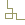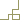• 关闭X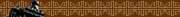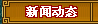欢迎大家光临易灵起名咨询馆·知命网。大家可以同时通过www.yiling.name或www.knowfate.net两个网址访问本站。本站站长易灵先生受民间顶级高人指点，是目前网络上唯一掌握了正确的命理理论、合婚理论、择日理论的人！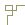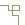易灵先生算八字聊天记录之二 发布者：易灵 发布时间：2016-07-15 18:26:11 阅读：76次 以下为易灵先生近日给四川某女士网上算命的聊天记录，为保护当事人隐私，名字已略去。希望对大家理解算命是怎么回事有所帮助。      f**(19:20:37): 您好, 在吗?    易灵(20:00:00): 你好，    f**(20:00:35): 您好, 我想请您帮我算一下. 可以吗?    f**(20:00:41): 是根据八字来算吗?    易灵(20:00:45): 对，   f**(20:02:41): 1978年7月18日(农历:6月14日) 下午5点半左右. 女.    易灵(20:02:59): 好的    f**(20:03:17): 过去, 现在, 将来的事, 您都会提到吗?    易灵(20:03:59): 对，   f**(20:06:57): 麻烦您开始算吧.    f**(20:07:06): 好的, 坏的, 都说. 不用忌讳. 呵呵    f**(20:07:09): 直言就可以了.    易灵(20:11:39): 好的，    易灵(20:12:17): 你的八字为戊午　已未　辛巳　丁酉    易灵(20:12:40): 另我想再问一下你父母和对象的属相    易灵(20:12:54): 因同年同月同日生的人命运不同是由于六亲不同引起的    f**(20:13:00): 嗯. 我爸属牛, 我妈属蛇.    f**(20:13:17): 对象, 接下来再算吧. 呵呵    易灵(20:14:28): 你父母是几月份知道吗    易灵(20:14:41): 因如果真是这两个属相的话，则你父母的婚配不好    f**(20:14:44): 我爸农历是二月.    f**(20:14:52): 我妈农历是十一月.    易灵(20:15:39): 哦，看来是这两个属相生的    易灵(20:15:48): 这么说你父母的婚配其实不算好    f**(20:15:56): 呵呵.您继续    易灵(20:16:00): 他们婚姻不和谐　    f**(20:16:04): 如果我有问题, 会提问您的.    f**(20:16:05): 哦.    易灵(20:16:06): 也就是说不到一起去    易灵(20:16:19): 而且有一个人身体不好    f**(20:16:39): 嗯. 我妈妈身体不太好.    易灵(20:16:53): 应该40多岁时就有反应了    易灵(20:17:06): 而且他们的感情也不算好，顶多是一般，    易灵(20:17:14): 有时还会有相互怀疑现象    易灵(20:17:18): 你是哪里人？    f**(20:17:23): 我啊, 四川人.    易灵(20:17:32): 哦，    易灵(20:17:51): 不过如果不计划生育的话，你父母也该生的女多男少    易灵(20:18:00): 现在计划生育，就不好说了    f**(20:18:11): 呵呵.    易灵(20:18:17): 你妈生的病也是慢性病，    f**(20:18:21): 您说的很细. 谢谢.    易灵(20:18:22): 很难好透    f**(20:18:26): 是的. 慢性病.    f**(20:18:31): 十几年了.    易灵(20:18:34): 该让她多注意身体，    f**(20:18:37): 我小学的时候, 她就生病了.    易灵(20:18:44): 而且今年身体还会有下降    f**(20:18:46): 嗯. 我刚给她买了保健品呢. 呵    易灵(20:18:53): 你是属于火命，    f**(20:18:54): 啊?还会下降吗    易灵(20:19:12): 嗯，总之她是慢性病，    易灵(20:19:26): 而且其实你爸可能有外遇的，    易灵(20:19:40): 只要平时多对父母好一些就可以了    易灵(20:19:48): 你是4岁生根，    易灵(20:19:57): 根生在钱柜下面    f**(20:20:15): 他是有外遇.    f**(20:20:25): 困扰我好多年了.    易灵(20:20:39): 嗯，父母的事情你不必多问的    f**(20:20:43): 根生在钱柜下面? 这是什么意思呢?    易灵(20:20:51): 就是好的意思。    易灵(20:21:13): 小时候相比其他小孩，你胆子还算大，不是很小的    易灵(20:21:34): 只是平时你跟你妈共同语言不多，特别是小时候每天都在一起的时候    易灵(20:21:41): 观念上易不一致    f**(20:22:02): 呵呵. 是的呢. 我妈好贤惠. 不过我和她的话题少. 现在好多了.比较能够理解她们了.    f**(20:22:16): 谢谢您算的这么细致啊    易灵(20:25:19): 你这个八字其实其他方面还算好，只是婚姻上会有些不太顺    易灵(20:25:28): 今年婚姻上不行，    易灵(20:25:34): 会与对象闹分手    f**(20:25:47): 哦. 会分吗? 还是闹分手呢?    易灵(20:26:00): 我需要知道另一位的属相    f**(20:26:06): 蛇    易灵(20:26:16): 一个巴掌拍不响的，所以需要知道双方属相    f**(20:26:39): 1977年10月6日(农历:8月24) 上午11点06分.    易灵(20:27:33): 唉，他这个八字今年也是不利婚，    f**(20:27:38): 哦.    易灵(20:27:39): 我看今年得分    f**(20:27:50): 呵呵,您的意思是, 我和他今年会分手?    易灵(20:28:12): 对，有这个可能    易灵(20:28:16): 不过话说回来，    易灵(20:28:34): 你跟他婚配其实还不差，    易灵(20:28:38): 只是今年流年不好，    f**(20:28:46): 不差的意思是?    易灵(20:28:52): 所以如果今年能克服一下，到明年就好了，    f**(20:29:05): 是的. 今年我和他的矛盾特别多.    f**(20:29:11): 我刚跟他提出分手了.    易灵(20:29:25): 所以这在于你自己把握了，你们的婚配还算好，    易灵(20:29:29): 能属于中等，    易灵(20:29:37): 关键是今年流年不好    f**(20:29:49): 哦. 中等啊?    f**(20:30:05): 也不算是很好的?    易灵(20:30:07): 现在走的大运和流年对你们的感情都有影响的    易灵(20:30:10): 对，中等，    f**(20:30:19): 就是平常的那种.    易灵(20:30:24): 婚配分上上、上中、上，中，下中，下下    易灵(20:30:34): 你们的婚配其实不是很差的那种    f**(20:30:35): 不会有太多波折?    易灵(20:30:44): 也会有些波折的，    f**(20:30:47): 呵呵, 如果很差的, 要来也没意思了.    易灵(20:31:01): 今年你们婚姻要经受很大的考验    易灵(20:31:10): 在农历十月份最厉害    f**(20:31:13): 其实中等, 我的理解就是, 不离婚的那种? 互相凑合着过.    f**(20:31:17): 最厉害?    易灵(20:31:20): 农历十月份从阳历11月8号开始    f**(20:31:23): 就是极有可能会分手?    易灵(20:31:40): 对，如果农历十月不离的话，则就可以克服过去了    易灵(20:31:56): 其实你是怄气跟他提出分手的    f**(20:31:57): 嗯.    易灵(20:32:06): 以前你对他分挺好的    f**(20:32:10): 怄气? 这是指?    易灵(20:32:29): 从命相上看，你对他的心不坏，    f**(20:32:39): 嗯.    易灵(20:32:43): 只不过你老公一直没走到什么好运    f**(20:32:51): 哦.    易灵(20:33:05): 到现在可以说一事无成，没有什么成就感    f**(20:33:14): 嗯.    易灵(20:33:42): 你老公一生中最好的运气在乙巳大运    f**(20:33:54): 那是多少岁的时候呢?    易灵(20:34:04): 上一步的丁未大运（1996年开始的十年）不    易灵(20:34:07): 不好    易灵(20:34:19): 从2006年开始的丙午大运也不好    易灵(20:34:33): 他可以说事业上很不顺    f**(20:34:50): 哦.    f**(20:34:57): 要多少年时, 可以顺些呢?    易灵(20:35:21): 不过从他命上看，其实还可以    易灵(20:35:25): 只是大运走得差了些    易灵(20:35:46): 他需从2017年往后，则一路好运    f**(20:36:05): 哦.    易灵(20:36:09): 不过从他命上看，应能有一个较好的单位    f**(20:36:11): 呵呵,还有十年呢    易灵(20:36:22): 对，十年后很好    易灵(20:36:47): 从去年开始的这步大运易破财之类    易灵(20:36:58): 今年还需防破财    f**(20:37:21): 哦.    f**(20:37:33): 那您觉得, 他会有婚外情吗?    易灵(20:37:46): 哦，你是怀疑他有婚外情呀    f**(20:37:55): 我现在没怀疑    f**(20:38:03): 我想请您算算, 他以后会有吗?    易灵(20:38:05): 从他运气看，现在没有    易灵(20:38:12): 以后也不太会有，    f**(20:38:16): 对, 现在没有的. 这我确定.    f**(20:38:51): 还没结婚呢.    易灵(20:39:00): 哦，    易灵(20:39:05): 这么大还没结婚呀，    f**(20:39:29): 呵呵.    f**(20:39:30): 是啊    f**(20:39:37): 所以您说我婚姻不顺, 是对的呢    易灵(20:40:02): 不过从他的八字看，该在2001年有至少是同居现象的    易灵(20:40:28): 所以你很可能不是他的第一个    f**(20:40:42): 他之前有两个正式的了    f**(20:40:47): 我是他第三任女朋友. 呵呵    易灵(20:40:53): 哦，    f**(20:40:56): 他没结过婚的.    易灵(20:41:19): 要是在过去，结婚就是同居了，    f**(20:41:24): 对头.呵    易灵(20:41:27): 现在社会开放，同居未必结婚的    f**(20:41:33): 是的.    易灵(20:41:39): 那你跟他是什么时候谈的？    f**(20:41:51): 他是01年大学毕业的. 那时他大学谈过一个女朋友.可能是那时吧    f**(20:42:00): 我啊, 今年才和他谈的.    易灵(20:42:16): 你去年也该谈过另一个的    f**(20:42:29): 太对了. 那个适合我吗?    易灵(20:42:49): 去年谈的那个，今年该分掉了    f**(20:43:08): 是的.    易灵(20:43:19): 2001年他已25岁了    f**(20:43:24): 我是2000年毕业.    f**(20:43:34): 他那时应该23岁吧.    f**(20:43:41): 他10月的生日呀    易灵(20:43:48): 我觉得要论工作单位，你们都还可以的    f**(20:43:54): 他高中复读了一年.    易灵(20:43:58): 应是管理性质的    f**(20:44:22): 哦.    易灵(20:44:24): 他1977年生，2001年不是25岁了呀    f**(20:44:32): 24岁不到.    易灵(20:45:16): 你在大学里应有人追求你的    f**(20:45:45): 对的    易灵(20:45:52): 其实你不是没谈，而是你不想谈    f**(20:45:58): 对了, 您看我的八字, 以后会离婚吗?    易灵(20:46:47): 是跟现在这个吗    f**(20:47:08): 嗯. 是吧    f**(20:47:21): 或者说, 您看我的八字里, 有没有带离婚的命格呢?    易灵(20:47:33): 还好啦，不是很明显    f**(20:47:42): 哦.没事的.    易灵(20:47:49): 不过还需看配什么样的老公    f**(20:47:50): 好的坏的都说.我不忌讳了.    f**(20:47:54): 哦.    f**(20:48:10): 那您看我和这个人, 会可能离婚吗? 假如以后能结婚的话.    易灵(20:48:48): 如果今年不分手的话，以后不太可能会离    易灵(20:48:53): 不过口角是难免的    f**(20:48:56): 嗯.    易灵(20:49:01): 也就是嘴上会斗    f**(20:49:03): 我和他的关系融洽吗? 以后    易灵(20:49:09): 不太融洽，    易灵(20:49:16): 但也不算很差    f**(20:49:19): 哦.    易灵(20:49:20): 中等吧    f**(20:49:23): 嗯.    易灵(20:49:27): 就是平平淡淡的那种    f**(20:49:30): 嗯.    易灵(20:49:36): 但比起你父母的那种婚配，则好了不少    f**(20:49:43): 就是说大家凑合一起. 但不会离婚. 呵    f**(20:49:54): 哦. 跟我父母的比,好在哪里呢?    易灵(20:50:28): 你想想你父母的婚配，一是你母亲身体不好，二是你爸有外遇，要你这事发生在你和你老公的身上，你会有何感觉    f**(20:50:46): 嗯. 就是说, 我不会像我母亲那样    易灵(20:50:50): 对，    f**(20:50:53): 他也不会有外遇?    易灵(20:50:56): 对，    易灵(20:51:03): 只是斗嘴而已    易灵(20:51:18): 明绞暗不绞的那种    f**(20:51:36): 哦. 暗不绞的意思是?    易灵(20:51:43): 就是命上不绞，    易灵(20:51:47): 只是嘴上绞    易灵(20:51:58): 今年也有可能你老公父亲的身体不好    f**(20:52:04): 嗯. 但是关系会不融洽, 就是不亲密?    f**(20:52:12): 哦. 他喜欢喝酒.    f**(20:52:21): 我觉得不好, 但也不好多劝.    易灵(20:52:30): 总之你们要是结婚也可以结，    f**(20:52:34): 嗯    易灵(20:52:42): 但也别指望有多恩爱，中等    f**(20:52:51): 嗯. 知道了    易灵(20:52:53): 不过平平淡淡才是真嘛    易灵(20:53:13): 现在你刚提出分手，我只怕到农历十月份你们会真的分手    f**(20:53:17): 我跟他的兴趣爱好, 还有朋友圈, 这些差异都很大.    f**(20:53:30): 哦. 真的分手的可能性很大?    f**(20:53:44): 不过现在也快到农历十月了.    易灵(20:53:51): 对，    f**(20:54:01): 所以估计可能性很大    易灵(20:54:03): 那分不分还在于你把握吧    f**(20:54:10): 在于我把握?    易灵(20:54:18): 嗯，我也不能劝你们分手呀，    f**(20:54:19): 他呢? 他也有决定权的呀.    易灵(20:54:31): 从他的八字看，今年也是不利于婚恋    易灵(20:54:38): 他今年会有失恋的可能    f**(20:54:39): 嗯.    f**(20:54:42): 哦.    易灵(20:54:49): 你们也可以在农历十月份少来往一点    f**(20:55:01): 现在已经不来往了. 呵呵    易灵(20:55:09): 哦，    f**(20:55:33): 那您看我的八字, 我以后会与婚姻吗? 就是会结婚吗?    易灵(20:56:01): 当然会结婚的    f**(20:56:07): 哦.    f**(20:56:17): 如果这个没成, 可能就会很晚了吧?    易灵(20:56:26): 嗯，    f**(20:56:38): 大概到多少岁呢    易灵(20:56:42): 不过你还是一切顺其自然吧   易灵(20:56:59): 如果真跟这个分手了，下次再谈时，注意有三个属相的不能碰    f**(20:57:02): 嗯. 我现在的心态就是顺其自然.    f**(20:57:08): 哦.哪三个呢    易灵(20:57:25): 牛，鼠，猴    易灵(20:57:28): 切记    f**(20:57:30): 哦.    f**(20:57:31): 好的.    易灵(20:57:38): 不过即使谈到其他属相的，最好也要合一下，    f**(20:57:43): 嗯.    易灵(20:57:44): 因为合婚在五个因素要考虑，    易灵(20:57:49): 属相只是其中之一    f**(20:57:53): 我下次再有, 会找您合的.    易灵(20:57:58): 好的，    易灵(20:58:10): 唉，你现在这个我也不好说你什么，    f**(20:58:18): 除了我的感情婚姻, 关于事业财运, 您说说吧.    易灵(20:58:23): 今年该有这么一劫，    f**(20:58:44): 嗯. 我觉得今年就是老想跟他闹别扭, 不想跟他一起了.    f**(20:58:53): 而且他的确也不太关心人那种.    易灵(20:58:57): 好的，婚姻就说这些吧    易灵(20:59:22): 你目前走的大运是丙辰，从工作的运气来看，总的还是好的    易灵(21:00:12): 用盲人的说法，你是进财命    f**(21:00:18): 哦.    易灵(21:00:21): 不象有的女的是退财命    f**(21:00:27): 财运很好?   吗?    易灵(21:00:57): 02年也可能跳了一个单位    易灵(21:01:09): 或者这年岗位上有变动的可能，    f**(21:01:13): 嗯.    易灵(21:01:20): 不过基本上向好的方向发展    f**(21:03:13): 嗯.是的    易灵(21:03:30): 跳后收入上也应有所增加    f**(21:03:47): 是的    易灵(21:04:02): 03－06年总的还不错    f**(21:04:29): 嗯.    易灵(21:04:51): 而且在这期间工作好象也不是很苦，有些管理的味道    f**(21:04:59): 是的    易灵(21:05:20): 今年从命上看，也有动象    f**(21:05:25): 嗯.    易灵(21:05:29): 一是婚姻上动，二是工作上动    易灵(21:05:36): 今年感觉没有前几年顺了    f**(21:05:40): 今年的动象, 有利于我事业以后的发展吗?    易灵(21:06:10): 从目前的大运看，还是好的，只不过流年稍差了些    f**(21:06:15): 哦.    f**(21:06:30): 那从哪年开始,我的事业运和财运会开始好起来呢    易灵(21:06:33): 不过你稍微克服一下就过去了    易灵(21:06:39): 从长远看，你是个富命    f**(21:06:58): 真的呀 太好了.    易灵(21:07:05): 从虚34岁往后，一路向富婆迈进了    易灵(21:07:19): 总体看，你的八字不错    f**(21:07:21): 就是2011年吧?    f**(21:07:27): 富婆的概念是?    易灵(21:07:30): 对    f**(21:07:36): 呵呵,多少钱算富婆呢?    易灵(21:07:43): 就是收入越来越好，财富越积越多的意思    f**(21:07:50): 嗯.    f**(21:08:00): 有多少级别, 您这里能看出来吗    f**(21:08:11): 因为, 现在说人富, 有很多说法    f**(21:08:16): 几十万也算可以    易灵(21:08:19): 你连续二十多年的财运    f**(21:08:24): 几百万也算富婆.    易灵(21:08:29): 几十万当然太少了    f**(21:08:31): 二十多年? 啊, 天啊.    f**(21:08:35): 哦. 几百万啊?    易灵(21:08:37): 至少几百万吧    f**(21:08:45): 哦.    易灵(21:08:49): 所以我说你是进财命    f**(21:08:52): 是靠我自己的能力挣的?    f**(21:09:01): 还是家里父母的呢?    易灵(21:09:13): 当然是靠自己    易灵(21:09:31): 不过你父母总的对你也不错的，对你命相有帮助    f**(21:09:45): 哦. 我的意思是, 是在我父母的公司, 还是在外面公司靠自己能力能挣这么多呢?    易灵(21:10:04): 不管在哪里，都会有这么多钱    易灵(21:10:15): 话说回来，你个人的能力也是不错的    f**(21:10:26): 哇. 真让我安慰    易灵(21:10:37): 如果是在父母的公司，你一定会把公司做得大很多    f**(21:10:47): 哈哈.    f**(21:10:50): 高兴哎.    f**(21:11:00): 我很希望能替我父母做点事.    易灵(21:11:02): 其实象你这么久的财运，我也是很少算到的    f**(21:11:27): 对了, 你能算出来, 我以后是更适于在哪里工作呢/ 是父母公司更好? 还是在外面公司   做?    f**(21:12:09): 您看我更适合在哪类企业里做呢? 谢谢.    易灵(21:12:31): 你爸的是私营企业吗    f**(21:12:40): 对的    f**(21:12:48): 以前是国营. 后来改制了    f**(21:12:59): 您太好了. 给我算这么多, 呵呵. 感谢!    易灵(21:13:07): 如果你在你爸的公司里做，也可以的，    f**(21:13:23): 哦. 但是我更适合哪个行业呢?    f**(21:13:35): 就是哪个行业更对我的事业发展有利    易灵(21:13:35): 与水有关的行业最好    f**(21:13:40): 水啊    易灵(21:13:42): 对，    f**(21:13:45): 没什么概念呢    易灵(21:14:06): 这种行业太多了，    f**(21:14:14): 哦. 比如说?    易灵(21:14:37): 你自己也可以想象一下的，只要沾水的就行    f**(21:14:46): 哦.那房地产肯定是不适合了. 呵呵    易灵(21:15:20): 对，房地产不太适合，不过关键你的大运好，即使做了也亏不了    f**(21:15:26): 呵呵.    易灵(21:15:41): 只不过没有发挥命运的最佳化罢了    f**(21:15:59): 是的.    易灵(21:15:59): 以后如果自己做老板了，最好也可以在风水上调理一下    易灵(21:16:08): 则可以发挥出最佳    f**(21:16:16): 到时候请您帮我看看. 当老板的那天.呵    易灵(21:16:30): 好呀，没问题的    易灵(21:16:37): 不过这几年你还得慢慢来呀    f**(21:16:47): 是啊. 要熬到2011年呢    f**(21:16:58): 其他方面, 我还顺利吗?    易灵(21:16:58): 你今年跳到什么单位去了？    f**(21:17:08): 也是制造型企业吧    f**(21:17:15): 不过换了一个工作类别.    易灵(21:17:18): 哦，与你爸的都有些相关呢    f**(21:17:24): 我以前学金融做投资的.    易灵(21:17:31): 那跳以前在什么单位呢    f**(21:17:33): 02-06年都做投资.    f**(21:17:41): 北京的一家大公司.    f**(21:17:45): 06年回XX,    f**(21:18:01): XX的金融投资环境不比北京.    f**(21:18:10): 我父亲也想我多接触机械类,    易灵(21:18:13): 那当然，毕竟是首都嘛    f**(21:18:15): 所以去的现在这家公司    f**(21:18:16): 对啊    易灵(21:18:27): 我有两个同学在XX的    f**(21:18:37): 呵呵.是吗.那以后有空来玩嘛    易灵(21:18:51): 好呀，肯定会过去的，我跟同学关系不错    易灵(21:19:22): 我觉得你以后很可能是个女老板，    易灵(21:19:27): 可以做些大事    f**(21:19:59): 哦.    f**(21:20:13): 呵呵, 承蒙您过奖了.    易灵(21:20:23): 以后干脆就接管你爸的事业吧，或者自己开创新的事情也行    易灵(21:20:32): 不过得等到下一步大运    易灵(21:20:37): 现在先积累经验吧    f**(21:20:49): 嗯. 好的.    f**(21:20:53): 我也这么想的    易灵(21:20:57): 你其他方面还好，没有什么不好    f**(21:21:07): 哦, 比如以后的身体健康什么啊?    f**(21:21:17): 要注意哪些方面呢    易灵(21:21:19): 身体没问题的    f**(21:21:29): 哦. 寿命在多少岁呢    易灵(21:21:32): 对了，以后做老板了，副手不可用属鼠的    f**(21:21:39): 哦. 好的.    易灵(21:21:40): 否则对你不忠心    f**(21:21:43): 好    易灵(21:21:58): 寿命长着呢    f**(21:21:59): 就是我身边的助手不能用属鼠的了    f**(21:22:02): 哦    易灵(21:22:06): 寿命不用担心的    易灵(21:22:16): 对，用这个属相不太好    f**(21:22:17): 嗯.    f**(21:22:22): 好的. 以后注意.    易灵(21:22:31): 其他方面没有要注意的了    f**(21:22:35): 嗯.    易灵(21:22:46): 对了，平时可以穿些深色的衣服，特别是以后自己开创事业时    f**(21:22:49): 我之前找人算过一个, 就是XX. 四川那个.    易灵(21:22:57): 哦，他算得准吗？    易灵(21:23:15): 我听说过XX    f**(21:23:25): 他说我有二十年大运, 到了快60岁时, 身体会有大问题. 如果能熬过那次, 就好些.    易灵(21:23:52): 算你过去的情况，你觉得他准确度有多少？    易灵(21:24:36): 哦，那总体上准确度百分之多少？    f**(21:24:54): 总体啊, 他说的没您细.    f**(21:25:15): 他说我命里缺水.    易灵(21:25:27): 对，所以我让你从事与水有关的最好    易灵(21:25:36): 或者平时多穿些深色的衣服    f**(21:25:38): 但他没说, 我和现在这个男朋友今年可能分手.    f**(21:25:52): 他说我和这个男朋友还不错, 比较配.    易灵(21:25:58): 哦，    易灵(21:26:08): 不管配还是不配，今年都有这一劫的    f**(21:26:13): 嗯.    f**(21:26:33): 对了. 那您能告诉我, 我命里的婚姻是哪年吗?    f**(21:26:40): 我爸妈给我压力蛮大的    f**(21:26:41): 唉    f**(21:26:52): 经常电话就说这个话题.    易灵(21:27:28): 目前的这步大运就有婚姻的    f**(21:27:47): 哦. 这步?是指哪年呢?    f**(21:27:57): 您说的一些术语,我不是很懂.    易灵(21:28:03): 这步大运到34岁结束    f**(21:28:30): 哦. 就是34以前能结婚了?    易灵(21:28:30): 09年很可能    f**(21:28:37): 哦.    易灵(21:28:51): 切记刚才的几个属相不要谈    易灵(21:28:57): 另外就是到时候最好再合一下    f**(21:29:06): 这步大运到34岁结束, 然后就是20多年财运了?    易灵(21:29:11): 很多不幸的婚姻都是由于婚配不好造成的    f**(21:29:15): 呵呵.好的. 到时候如果换了, 再请您算.    易灵(21:29:16): 对，    f**(21:29:28): 我感觉这个告吹的可能性很大.    f**(21:29:35): 我们已经好几天没联系了.    f**(21:29:50): 他的性格也属于比较刚硬的那种.    f**(21:30:24): 对了, 您顺便能帮我看看那个男孩的感情婚姻情况吗?    f**(21:31:01): 其实我对他事业好不好有没有钱不在意的.    f**(21:31:14): 关键我觉得他的性格比较粗线条, 不太会关心人. [返回上一页]